# Koch snowflake

Koch snowflake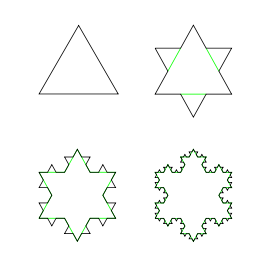The first four iterations of the Koch snowflakeThe first seven iterations in animationThe Koch curve

The Koch snowflake (also known as the Koch star and Koch island) is a mathematical curve and one of the earliest fractal curves to have been described. It is based on the Koch curve, which appeared in a 1904 paper titled "On a continuous curve without tangents, constructible from elementary geometry" (original French title: "Sur une courbe continue sans tangente, obtenue par une construction géométrique élémentaire") by the Swedish mathematician Helge von Koch.

## Construction

The Koch snowflake can be constructed by starting with an equilateral triangle, then recursively altering each line segment as follows:

1. divide the line segment into three segments of equal length.
2. draw an equilateral triangle that has the middle segment from step 1 as its base and points outward.
3. remove the line segment that is the base of the triangle from step 2.

After one iteration of this process, the result is a shape similar to the Star of David.

The Koch snowflake is the limit approached as the above steps are followed over and over again. The Koch curve originally described by Koch is constructed with only one of the three sides of the original triangle. In other words, three Koch curves make a Koch snowflake.

## Properties

The Koch curve has an infinite length because each time the steps above are performed on each line segment of the figure there are four times as many line segments, the length of each being one-third the length of the segments in the previous stage. Hence, the total length increases by one third and thus the length at step n will be (4/3)n of the original triangle perimeter: the fractal dimension is log 4/log 3 ≈ 1.26, greater than the dimension of a line (1) but less than Peano's space-filling curve (2).

The Koch curve is continuous everywhere but differentiable nowhere.

Taking s as the side length, the original triangle area is$\frac{s^2\sqrt{3}}{4}$ . The side length of each successive small triangle is 1/3 of those in the previous iteration; because the area of the added triangles is proportional to the square of its side length, the area of each triangle added in the nth step is 1/9 of that in the (n-1)th step. In each iteration after the first, 4 times as many triangles are added as in the previous iteration; because the first iteration adds 3 triangles then the nth iteration will add$3 \cdot 4^{n-1}$ triangles. Combining these two formulae gives the iteration formula:$A_{n+1} = A_n + \frac{3 \cdot 4^{n-1}}{9^n}A_0\quad n \ge 1 \, ,$

where A0 is area of the original triangle. Substituting in$A_1 = \frac{4}{3} A_0 \, ,$

and expanding yields:$A_{n+1} = \frac{4}{3} A_0 + \sum_{k=2}^n \frac{3 \cdot 4^{k-1}}{9^k} A_0 = (\frac{4}{3} + \frac{1}{3} \sum_{k=2}^n 3\frac{3 \cdot 4^{k-1}}{9^k}) A_0 =(\frac{4}{3} + \frac{1}{3} \sum_{k=2}^n \frac{9 \cdot 4^{k-1}}{9^k}) A_0 = \left (\frac{4}{3} + \frac{1}{3} \sum_{k=1}^n \frac{4^{k}}{9^k} \right ) A_0 \, .$

In the limit, as n goes to infinity, the limit of the sum of the powers of 4/9 is 4/5, so$\lim_{n \rightarrow \infty} A_n = \left( \frac{4}{3} + \frac{1}{3} \cdot \frac{4}{5} \right ) A_0 = \frac{8}{5} A_0 \, .$

So the area of a Koch snowflake is 8/5 of the area of the original triangle, or$\frac{2s^2\sqrt{3}}{5}$. Therefore the infinite perimeter of the Koch triangle encloses a finite area.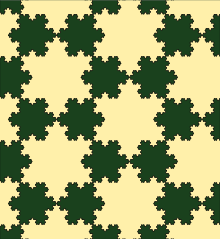Tesselation by two sizes of Koch snowflake

It is possible to tessellate the plane by copies of Koch snowflakes in two different sizes. However, such a tessellation is not possible using only snowflakes of the same size as each other. Since each Koch snowflake in the tesselation can be subdivided into seven smaller snowflakes of two different sizes, it is also possible to find tessellations that use more than two sizes at once.

## Thue-Morse Sequence and Turtle graphics

A Turtle Graphic is the curve that is generated if an automaton is programmed with a sequence. If the Thue–Morse sequence members are used in order to select program states:

• If t(n) = 0, move ahead by one unit,
• If t(n) = 1, rotate counterclockwise by an angle of π/3,

the resulting curve converges to the Koch snowflake.

## Representation as Lindenmayer system

The Koch Curve can be expressed by a rewrite system (Lindenmayer system).

Alphabet : F
Constants : +, −
Axiom : F++F++F
Production rules:
F → F−F++F−F

Here, F means "draw forward", + means "turn right 60°", and means "turn left 60°".

## Variants of the Koch curve

Following von Koch's concept, several variants of the Koch curve were designed, considering right angles (quadratic), other angles (Césaro) or circles and their extensions to higher dimensions (Sphereflake):

Variant Illustration Construction
1D, 85° angleCesaro fractal
The Cesaro fractal is a variant of the Koch curve with an angle between 60° and 90° (here 85°).
1D, 90° angle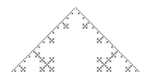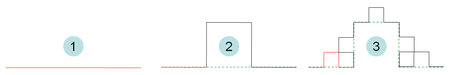The first 2 iterations
1D, 90° angle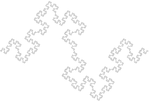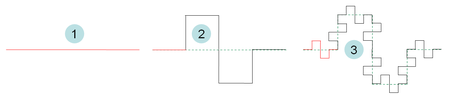The first 2 iterations. Its fractal dimension equals 1.5 and is exactly half-way between dimension 1 and 2. It is therefore often chosen when studying the physical properties of non-integer fractal objects.
2D, triangles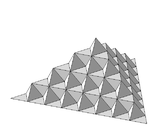von Koch surface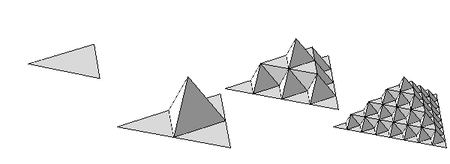The first 3 iterations of a natural extension of the Koch curve in 2 dimensions
2D, 90° angle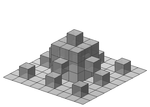Extension of the quadratic type 1 curve. The illustration at left shows the fractal after the second iteration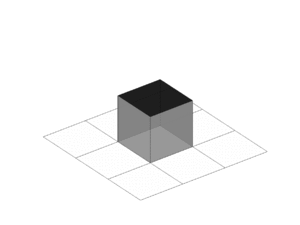.
2D, 90° angle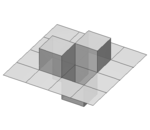Extension of the quadratic type 2 curve. The illustration at left shows the fractal after the first iteration.
2D, spheresCloseup of Haines sphereflake
Eric Haines has developed the sphereflake fractal, which is a three-dimensional version of the Koch snowflake, using spheres.

## Notes and references

1. ^ Addison, Paul S. Fractals and Chaos - An Illustrated Course. Institute of Physics (IoP) Publishing (1997) ISBN: 0750304006 - Page 19
2. ^ Koch Snowflake
3. ^ Burns, Aidan (1994), "78.13 Fractal tilings", Mathematical Gazette 78 (482): 193–196, JSTOR 3618577 .

Wikimedia Foundation. 2010.

### Look at other dictionaries:

• Snowflake — may refer to:* A particle of snow * Snowflake (albino gorilla) * Snowflake (plant), flowering plants in the genus Leucojum * Snowflake, Arizona * Snowflake schema, a concept in data management * Snowflake children, a child conceived through in… …   Wikipedia

• Koch (surname) — Koch (pronounced in German, although it is often pronounced differently in other countries) is a German surname meaning cook and may refer to: * Bill Koch (skier), cross country skier * Billy Koch, Major league baseball relief pitcher * Bobby… …   Wikipedia

• Koch — may refer to: * Koch (surname), people with this surname * Koch (boat), a type of Arctic boat * Koch (language), a language spoken in India and Bangladesh * Koch method of learning Morse Code * Koch snowflake or star, and Koch curve * Koch s… …   Wikipedia

• Koch, Niels Fabian Helge von — ▪ Swedish mathematician born January 25, 1870, Stockholm, Sweden died March 11, 1924, Stockholm  Swedish mathematician famous for his discovery of the von Koch snowflake curve, a continuous curve important in the study of fractal geometry.… …   Universalium

• Koch-Kurve — Kochsche Schneeflocke, Konstruktion. Die Koch Kurve oder kochsche Kurve ist ein von dem schwedischen Mathematiker Helge von Koch 1904 vorgestelltes Beispiel für eine überall stetige, aber nirgends differenzierbare Kurve. Es handelt sich bei ihr… …   Deutsch Wikipedia

• Koch'sche Schneeflocke — Die Koch Kurve oder kochsche Kurve ist ein von dem schwedischen Mathematiker Helge von Koch 1904 vorgestelltes Beispiel für eine überall stetige, aber nirgends differenzierbare Kurve. Es handelt sich bei ihr ferner um eines der ersten formal… …   Deutsch Wikipedia

• Copo de nieve de Koch — Saltar a navegación, búsqueda Concepción artística de un Copo de Koch El copo de nieve de Koch es una de las más sencillas figuras fractales, y una de las primeras. Fue inventada por el matemático sueco Helge von Koch …   Wikipedia Español

• Helge von Koch — Niels Fabian Helge von Koch (January 25, 1870 March 11, 1924) was a Swedish mathematician, who gave his name to the famous fractal known as the Koch snowflake, which was one of the earliest fractal curves to have been described.He was born into a …   Wikipedia

• Fractal — A fractal is generally a rough or fragmented geometric shape that can be split into parts, each of which is (at least approximately) a reduced size copy of the whole, [cite book last = Mandelbrot first = B.B. title = The Fractal Geometry of… …   Wikipedia

• Geometric series — In mathematics, a geometric series is a series with a constant ratio between successive terms. For example, the series:frac{1}{2} ,+, frac{1}{4} ,+, frac{1}{8} ,+, frac{1}{16} ,+, cdotsis geometric, because each term is equal to half of the… …   Wikipedia